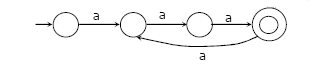Related Articles

# Automata Theory | Set 4

• Difficulty Level : Expert
• Last Updated : 27 Mar, 2017

Following questions have been asked in GATE CS 2011 exam.

1) Let P be a regular language and Q be context-free language such that Q ⊆ P. (For example, let P be the language represented by the regular expression p*q* and Q be {pnqn|n ∈ N}). Then which of the following is ALWAYS regular?
(A) P ∩ Q
(B) P – Q
(C) ∑* – P
(D) ∑* – Q

The expression ∑* – P represents complement of P which is a regular language. Complement of Regular languages is also regular. Then a DFA that accepts the complement of L, i.e. ∑* – L, can be obtained by swapping its accepting states with its non-accepting states.

2) Consider the language L1,L2,L3 as given below.
L1={0p1q | p,q ∈ N}
L2={0p1q| p,q ∈ N and p=q}
L3={0p1q0r | p,q,r ∈N and p=q=r}
Which of the following statements is NOT TRUE?

(A) Push Down Automata (PDA) can be used to recognize L1 and L2
(B) L1 is a regular language
(C) All the three languages are context free
(D) Turing machine can be used to recognize all the three languages

The language L3 is not context free. Refer this for more details.

3)Definition of a language L with alphabet {a} is given as following. L= { ank | k > 0, and n is a positive integer constant} What is the minimum number of states needed in a DFA to recognize L?
(A) k+1
(B) n+1
(C) 2n+1
(D) 2k+1